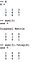# Image Processing using Matlab/Octave — Part 1

This will a multipart series on image processing using Matlab/Octave in which we’ll learn about images, basics of Matlab/Octave, using different filters on images, converting images into different colour models etc.

So, what is Image Processing ? Precisely Digital Image Processing

The field of digital image processing refers to processing of digital images by means of a digital computer. — Digital Image Processing (Gonzalez & Woods)

Digital image processing is a sub field of digital signal processing.

# How image processing works ?

World around us → Captured by Camera → Computer processes it → Output is a processed image

We capture photos, store on our devices, load and edit in softwares and then the image is ready. Softwares such as Adobe Photoshop and apps like Snapseed etc. use various efficient algorithms to modify image, like the one below. The image before looks blurry when it was captured but after processing it the image looks really nice. This is image processing.

# What is an Image ?

Now lets talk about what an image is.

An image is nothing but a 2-D matrix(grayscale) or 3-D matrix(coloured) and is represented by a mathematical function f(x,y), where x and y are the two co-ordinates horizontally and vertically.

What is a matrix ?

The is a square matrix in which the horizontal arrangement of numbers is called rows and the vertical arrangement is called columns. An image is the same as a matrix, but the numbers filling it are the intensities.

The smallest possible addressable element in an image is called pixel. The intensity is the representation of the value of the pixel in an image. So, a MxN image tells us that there are M rows and N columns and there are MN pixels.

So, what is this intensity value ? This value lies in the range 0–255 where 0 represents black and 255 means white for a grayscale image.

A grayscale image has only 1 channel, whereas a coloured image has 3 channels i.e., three 2-D matrices stacked on top of each other.

In a grayscale image a single pixel is represented by 256 unique values (0–255), or 2⁸ unique values. This 8 represents the bpp or bits per pixel i.e., the number of bits required to represent a pixel.

Similarly for a coloured image, as there are 3 channels and each pixel in a single channel is represented by 8 bits then then total bits required to represent a single pixel in RGB image will be 8+8+8 = 24 bits i.e., 16.7 million colours represented by a single pixel whereas in a grayscale image 256 colours.

The RGB stands for Red Green Blue which is an additive colour model in which the red, green and blue are added together in various ways to reproduce a broad array of colours.

The size of image is calculated as rows*columns*bpp, eg: a 100x100 grayscale image has size: 100*100*8 = 80,000 bits and converting it into bytes 80000/1024 = 78.12 bytes i.e., it will acquire 78.12 bytes for storage.

Storing of images is done in the 3-D format (rows, columns, channels). Sometimes in machine learning the number of channels is put before rows like (channels, rows, columns).

There is one more type of image called Binary image which is represented by only two values - 0 and 1, 0 for black and 1 for white.

We will be working on Octave/Matlab, depends on your choice. Both have nearly the same functionality.Octave is a free software whereas Matlab is paid. I’ll be using Octave 😉 .

# Basic operations in Octave/Matlab

The basic operations such as +, -, /, *, %, <, > etc. works the same as in most of the programming languages.

A matrix is defined as follows:

A 2-D matrix is defined by adding semicolons:

What about 3-D matrix ? First we’ll define a 2-D matrix and then add depth to it.The format is (rows, columns, depth). For an RGB image the depth is 3.

Now let’s see some operations on matrices:

Subtraction:

Multiplication:

Here B’ is the transpose of B, i.e., B’ has dimension 4x2.

Element wise multiplication:

. is used for doing element-wise calculations. It can used as ./ for doing element-wise division.

Following are some more operations:

For creating diagonal matrix we’ll use eye() and diag() functions:eye(3) creates a 3x3 identity matrix and diag(X) gets diagonal elements from X

# Accessing elements in a matrix:

Note: Indexing in Matlab/Octave starts with 1 rather than 0

For accessing elements just specify the row and column number.

We can also specify range, using colon.The below can be read as get elements from row 1 to 2 and column 1 to 2 from X.

For getting specific rows or columns, define matrix of the ones you want to access. The below can be read as, get elements of row 1 and 3 and column 2 from X.

That’s all. Now you know the basic of Matlab/Octave. We’ll use these concepts in image processing in the next part of this story.

I hope you liked the content.

Best of luck and keep practicing 😄.

Machine/Deep Learning, Amateur Photographer